# Tricomi equation

(diff) ← Older revision | Latest revision (diff) | Newer revision → (diff)

A differential equation of the form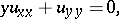which is a simple model of a second-order partial differential equation of mixed elliptic-hyperbolic type with two independent variablesand one open non-characteristic interval of parabolic degeneracy. The Tricomi equation is elliptic for, hyperbolic forand degenerates to an equation of parabolic type on the line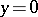(see ). The Tricomi equation is a prototype of the Chaplygin equation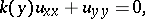where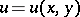is the stream function of a plane-parallel steady-state gas flow,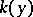andare functions of the velocity of the flow, which are positive at subsonic and negative at supersonic speeds, andis the angle of inclination of the velocity vector (see  ).

Many important problems in the mechanics of continuous media reduce to a boundary value problem for the Tricomi equation, in particular, mixed flows involving the formation of local subsonic zones (see , ).

How to Cite This Entry:
Tricomi equation. Encyclopedia of Mathematics. URL: http://encyclopediaofmath.org/index.php?title=Tricomi_equation&oldid=17070
This article was adapted from an original article by A.M. Nakhushev (originator), which appeared in Encyclopedia of Mathematics - ISBN 1402006098. See original article